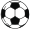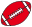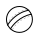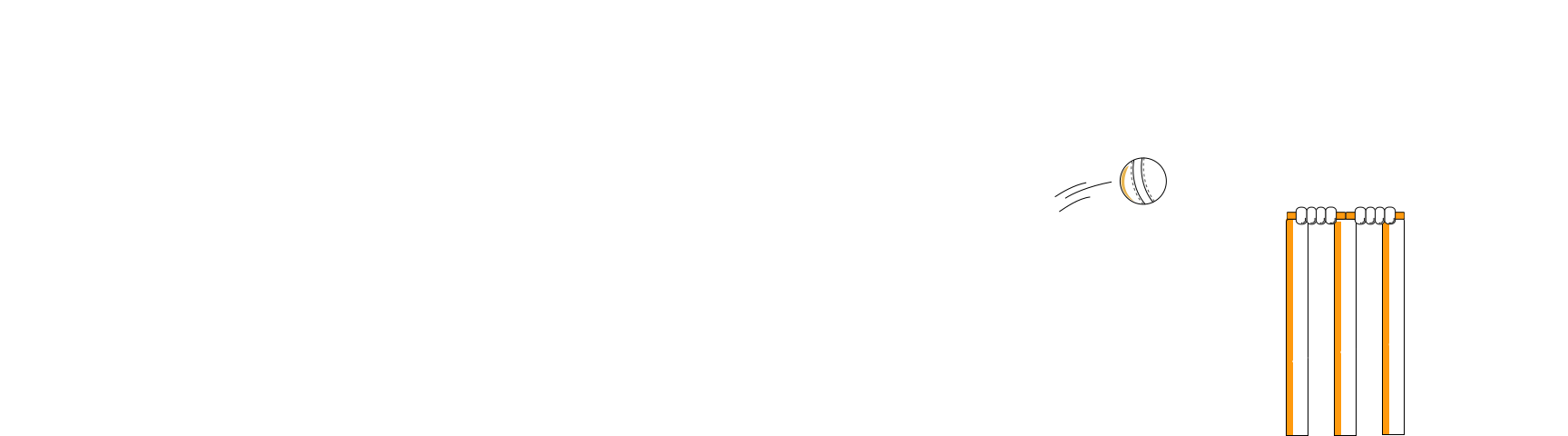🏏 450 million fans watched the last IPL. What is 'Cricket Scale' for SREs? Know More

## What in the world is Cricket Scale?self.__wrap_b=(o,n,e)=>{e=e||document.querySelector(`[data-br="\${o}"]`);let a=e.parentElement,i=_=>e.style.maxWidth=_+"px";e.style.maxWidth="";let t=a.clientWidth,c=a.clientHeight,s=t/2-.25,l=t+.5,u;if(t){for(;s+1<l;)u=Math.round((s+l)/2),i(u),a.clientHeight===c?l=u:s=u;i(l*n+t*(1-n))}e.__wrap_o||(typeof ResizeObserver!="undefined"?(e.__wrap_o=new ResizeObserver(()=>{self.__wrap_b(0,+e.dataset.brr,e)})).observe(a): false&&0)};self.__wrap_b(":R18p:",1)

There are only a few events in the world that attract a global audience.Half the world watched the 2022 FIFA World Cup.113MPeople watched the Superbowl.And then, there’s Cricket!450MPeople watched the last big cricketing event; the Indian Premier League.30MHighest concurrent viewers ever on an OTT platform.

## What does this mean?self.__wrap_b=(o,n,e)=>{e=e||document.querySelector(`[data-br="\${o}"]`);let a=e.parentElement,i=_=>e.style.maxWidth=_+"px";e.style.maxWidth="";let t=a.clientWidth,c=a.clientHeight,s=t/2-.25,l=t+.5,u;if(t){for(;s+1<l;)u=Math.round((s+l)/2),i(u),a.clientHeight===c?l=u:s=u;i(l*n+t*(1-n))}e.__wrap_o||(typeof ResizeObserver!="undefined"?(e.__wrap_o=new ResizeObserver(()=>{self.__wrap_b(0,+e.dataset.brr,e)})).observe(a): false&&0)};self.__wrap_b(":R1hp:",1)

This is a BIG deal for a Site Reliability Engineers.

The team has to prepare for tackling unknowns at such an unprecedented scale.“Observability is essential for modern software and can bring significant benefits. However, it is challenging to implement correctly. Last9's founders have been valuable co-build partners in creating a robust observability platform that covers all the necessary building blocks.”

Akash Saxena

ex-CTO at Disney+ Hotstar

## How crazy do things get?self.__wrap_b=(o,n,e)=>{e=e||document.querySelector(`[data-br="\${o}"]`);let a=e.parentElement,i=_=>e.style.maxWidth=_+"px";e.style.maxWidth="";let t=a.clientWidth,c=a.clientHeight,s=t/2-.25,l=t+.5,u;if(t){for(;s+1<l;)u=Math.round((s+l)/2),i(u),a.clientHeight===c?l=u:s=u;i(l*n+t*(1-n))}e.__wrap_o||(typeof ResizeObserver!="undefined"?(e.__wrap_o=new ResizeObserver(()=>{self.__wrap_b(0,+e.dataset.brr,e)})).observe(a): false&&0)};self.__wrap_b(":Rap:",1)

### Think 600+ million metrics a minute at 200 ms of ingestion.self.__wrap_b=(o,n,e)=>{e=e||document.querySelector(`[data-br="\${o}"]`);let a=e.parentElement,i=_=>e.style.maxWidth=_+"px";e.style.maxWidth="";let t=a.clientWidth,c=a.clientHeight,s=t/2-.25,l=t+.5,u;if(t){for(;s+1<l;)u=Math.round((s+l)/2),i(u),a.clientHeight===c?l=u:s=u;i(l*n+t*(1-n))}e.__wrap_o||(typeof ResizeObserver!="undefined"?(e.__wrap_o=new ResizeObserver(()=>{self.__wrap_b(0,+e.dataset.brr,e)})).observe(a): false&&0)};self.__wrap_b(":Rqp:",1)

• Chaotic war rooms

• Crashing dashboards due to Concurrent access

• Static alerting not keeping up with scale

• Unstable Observability Infrastructure

There are no playbooks on how to serve that scale.## Understanding Customer Patternsself.__wrap_b=(o,n,e)=>{e=e||document.querySelector(`[data-br="\${o}"]`);let a=e.parentElement,i=_=>e.style.maxWidth=_+"px";e.style.maxWidth="";let t=a.clientWidth,c=a.clientHeight,s=t/2-.25,l=t+.5,u;if(t){for(;s+1<l;)u=Math.round((s+l)/2),i(u),a.clientHeight===c?l=u:s=u;i(l*n+t*(1-n))}e.__wrap_o||(typeof ResizeObserver!="undefined"?(e.__wrap_o=new ResizeObserver(()=>{self.__wrap_b(0,+e.dataset.brr,e)})).observe(a): false&&0)};self.__wrap_b(":R5ip:",1)

Engineers need a precise vocabulary to talk to a system, and spot customer patterns.This vocabulary helps detect when something is not right, and where things are not right.“Our global infrastructure generates millions of events per minute, which we ingest into Last9 for analysis, monitoring and alerting.This thing scales.

Subhash Choudhary

Co-Founder & CTO

## How do SREs manage Cricket scale?self.__wrap_b=(o,n,e)=>{e=e||document.querySelector(`[data-br="\${o}"]`);let a=e.parentElement,i=_=>e.style.maxWidth=_+"px";e.style.maxWidth="";let t=a.clientWidth,c=a.clientHeight,s=t/2-.25,l=t+.5,u;if(t){for(;s+1<l;)u=Math.round((s+l)/2),i(u),a.clientHeight===c?l=u:s=u;i(l*n+t*(1-n))}e.__wrap_o||(typeof ResizeObserver!="undefined"?(e.__wrap_o=new ResizeObserver(()=>{self.__wrap_b(0,+e.dataset.brr,e)})).observe(a): false&&0)};self.__wrap_b(":R1bp:",1)

1

Instrumentation

2

External Dependencies

Map out External Dependencies such as CDN configurations, 3rd-party APIs and Payment services.

3

Identify Metrics

Identify key critical metrics that can give early warnings.

4

SLOs

Understand latencies and availability baselines to write SLOs that matter.

These are the absolute four basics that need to come together days before the match starts so one can load test and prepare for the Cricket scale.

#### Want to know how we help teams prepare for Cricket Scale?self.__wrap_b=(o,n,e)=>{e=e||document.querySelector(`[data-br="\${o}"]`);let a=e.parentElement,i=_=>e.style.maxWidth=_+"px";e.style.maxWidth="";let t=a.clientWidth,c=a.clientHeight,s=t/2-.25,l=t+.5,u;if(t){for(;s+1<l;)u=Math.round((s+l)/2),i(u),a.clientHeight===c?l=u:s=u;i(l*n+t*(1-n))}e.__wrap_o||(typeof ResizeObserver!="undefined"?(e.__wrap_o=new ResizeObserver(()=>{self.__wrap_b(0,+e.dataset.brr,e)})).observe(a): false&&0)};self.__wrap_b(":Rjbp:",1)

Slide into our DMs## What makes Last9 special?self.__wrap_b=(o,n,e)=>{e=e||document.querySelector(`[data-br="\${o}"]`);let a=e.parentElement,i=_=>e.style.maxWidth=_+"px";e.style.maxWidth="";let t=a.clientWidth,c=a.clientHeight,s=t/2-.25,l=t+.5,u;if(t){for(;s+1<l;)u=Math.round((s+l)/2),i(u),a.clientHeight===c?l=u:s=u;i(l*n+t*(1-n))}e.__wrap_o||(typeof ResizeObserver!="undefined"?(e.__wrap_o=new ResizeObserver(()=>{self.__wrap_b(0,+e.dataset.brr,e)})).observe(a): false&&0)};self.__wrap_b(":Rc9:",1)

### We have Levitate. A Managed Time Series Data Warehouse.self.__wrap_b=(o,n,e)=>{e=e||document.querySelector(`[data-br="\${o}"]`);let a=e.parentElement,i=_=>e.style.maxWidth=_+"px";e.style.maxWidth="";let t=a.clientWidth,c=a.clientHeight,s=t/2-.25,l=t+.5,u;if(t){for(;s+1<l;)u=Math.round((s+l)/2),i(u),a.clientHeight===c?l=u:s=u;i(l*n+t*(1-n))}e.__wrap_o||(typeof ResizeObserver!="undefined"?(e.__wrap_o=new ResizeObserver(()=>{self.__wrap_b(0,+e.dataset.brr,e)})).observe(a): false&&0)};self.__wrap_b(":Rk9:",1)Levitate is built from the ground up with warehousing capabilities.

• Scale Reliably
• Slash Costs
• Tame High Cardinality

Open Standards We are Prometheus and OpenTelemetry compatible, and work with most Open Standard protocols.

High Cardinality Control data growth with streaming aggregations and slash operational overhead of expensive queries.

Data Tiering Incoming data is automatically tiered to optimize time series storage based on specific metrics use cases.

50% Less TCO Levitate is available in two models: SaaS and Managed Deployment. With the Managed Deployment option, eliminate engineering toil and hidden egress data transfer costs.

Global Availability Available across geographies so data never leaves your infrastructure. Levitate commits to a 99.9% write-availability and a 99.5% read-availability.## How does this help manage Cricket Scale?self.__wrap_b=(o,n,e)=>{e=e||document.querySelector(`[data-br="\${o}"]`);let a=e.parentElement,i=_=>e.style.maxWidth=_+"px";e.style.maxWidth="";let t=a.clientWidth,c=a.clientHeight,s=t/2-.25,l=t+.5,u;if(t){for(;s+1<l;)u=Math.round((s+l)/2),i(u),a.clientHeight===c?l=u:s=u;i(l*n+t*(1-n))}e.__wrap_o||(typeof ResizeObserver!="undefined"?(e.__wrap_o=new ResizeObserver(()=>{self.__wrap_b(0,+e.dataset.brr,e)})).observe(a): false&&0)};self.__wrap_b(":R1c9:",1)

Levitate is the Time Series Data Warehouse that SREs trust.Key numbers from the last IPL.

#### Case Studyself.__wrap_b=(o,n,e)=>{e=e||document.querySelector(`[data-br="\${o}"]`);let a=e.parentElement,i=_=>e.style.maxWidth=_+"px";e.style.maxWidth="";let t=a.clientWidth,c=a.clientHeight,s=t/2-.25,l=t+.5,u;if(t){for(;s+1<l;)u=Math.round((s+l)/2),i(u),a.clientHeight===c?l=u:s=u;i(l*n+t*(1-n))}e.__wrap_o||(typeof ResizeObserver!="undefined"?(e.__wrap_o=new ResizeObserver(()=>{self.__wrap_b(0,+e.dataset.brr,e)})).observe(a): false&&0)};self.__wrap_b(":R249:",1)India vs Pakistan, Site Reliability Engineering, and Shannon Limit

Customer Success, Last9

## Our goal

### Making Observability a first-class citizen so you can build your reliability to the last 9.self.__wrap_b=(o,n,e)=>{e=e||document.querySelector(`[data-br="\${o}"]`);let a=e.parentElement,i=_=>e.style.maxWidth=_+"px";e.style.maxWidth="";let t=a.clientWidth,c=a.clientHeight,s=t/2-.25,l=t+.5,u;if(t){for(;s+1<l;)u=Math.round((s+l)/2),i(u),a.clientHeight===c?l=u:s=u;i(l*n+t*(1-n))}e.__wrap_o||(typeof ResizeObserver!="undefined"?(e.__wrap_o=new ResizeObserver(()=>{self.__wrap_b(0,+e.dataset.brr,e)})).observe(a): false&&0)};self.__wrap_b(":R2cp:",1)

Talk to usCloud Native Monitoring